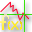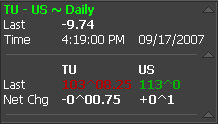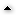##Spread Charts

"Spread Charts" helps you to watch over the evolution of a contract in comparison with another one. A simple spread chart can be, for example, the price of the first contract minus the price of the second contract. In the example below there are two contracts: TU and US with the multipliers: 1 and -1.

The price for the "Spread Chart" is computed using formula:

Spread Chart = a * X + b * Y

where:

 a = the multiplier for the first contract (1) b = the multiplier for the second contract (-1) X = the close price for the first contract (TU) Y = the close price for the second contract (US)If you don't want to use contracts with multipliers you can write your own formula. See how a new spread chart is created.